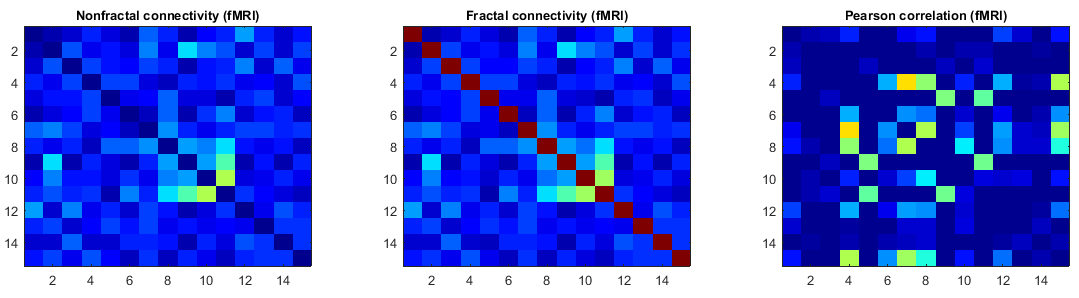# nonfractal: MATLAB Toolbox for estimating nonfractal connectivity and fractal connectivity

## Description

It is a MATLAB toolbox for estimating both nonfractal connectivity and fractal connectivity from a set of time series with long-range dependence such as resting state fMRI BOLD signals.

## Depends

• Statistics Toolbox
• wmtsa

## Author

Wonsang You ([email protected])

## Getting Started

This toolbox was designed to work from MATLAB version 7.7 (R2008b) to the latest version with the Statistics toolbox. You can install this toolbox as follows.

1. Place all files of this toolbox at any directory, and add all sub-directories to the MATLAB paths.
3. Try to run the demo script "`bfn_demo_nonfractal`". This demo shows an example of estimating nonfractal connectivity of resting state fMRI BOLD signals of the rat brain using the maximum likelihood estimator.## Functions

Let X be a NxQ matrix of Q time series with length N. It might be a set of BOLD signals corresponding to multiple ROIs of the brain which was extracted from DICOM images. Then, the function "`bfn_mfin_ml`" estimates the Hurst exponent, nonfractal connectivity, and fractal connectivity in multivariate time series with long memory using the maximum likelihood method as follows.

[H, nfcon, fcon] = bfn_mfin_ml(X);

H is the Hurst exponent, nfcon and fcon denote the nonfractal connectivity and fractal connectivity respectively. Nonfractal connectivity is identical to the correlation matrix of short memory while fractal connectivity is defined as the asymptotic wavelet correlation of bivariate long memory processes. On the other hand, the function "`bfn_mfin_lms`" uses the least-mean squares (LMS) method to estimate all the above parameters.

[H, nfcon, fcon] = bfn_mfin_lms(X);

For more options, run the commands "`help bfn_mfin_ml`" or "`help bfn_mfin_lms`".

## Reference

Please cite the following paper when using this toolbox.

Wonsang You, Sophie Achard, Joerg Stadler, Bernd Bruekner, and Udo Seiffert, "Fractal analysis of resting state functional connectivity of the brain," in 2012 International Joint Conference on Neural Networks, 2012.

## Technical supports

Get A Weekly Email With Trending Projects For These Topics
No Spam. Unsubscribe easily at any time.
Matlab (30,882
Statistics (10,410
Time Series (4,057
Connectivity (1,774
Fractal (1,771
Neuroimaging (611
Fmri (196
Matlab Toolbox (115
Statistics Toolbox (8
Resting State (6
Hurst Parameter (5